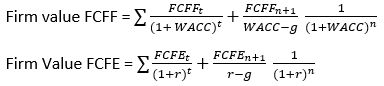# CFA Level II: Equity Valuation – FCFF and FCFE Concepts

FCFF/FCFE Concepts

A common equity valuation method is the discounted FCFF or FCFE method. FCFF is free cash flow to firm and FCFE is free cash flow to equity.

The valuation method is similar for both:For two stage or multistage models, we use a similar equation but incorporate a present value calculation for each stage.Notice that FCFF uses WACC as its discount rate because cost of debt is no accounted for in the FCFF. Debt is incorporated into the FCFE calculation, so we use the cost of equity as that discount rate. Because of this, leverage changes do not affect FCFF calculations and minimal effect on FCFE calculations.

Dividends, share repurchases, and shares issues have no effect of FCFF or FCFE.

The core concept is to understand how to calculate FCFF and FCFE, given the variety of ways we can derive each from the balance sheet and income statement. Broadly speaking, FCFF is the cash that is available to investors and bondholders after cash operating expenses and short and long-term investments are taken out of revenues. FCFE goes down a level by removing payments to bondholders from FCFF, resulting in the amount of cash that is available after all obligations have been met.

FCFF Calculations:

• FCFF = NI + NCC + Int(1 – Tax rate) – FCInv – WCInv
• FCFF = CFO + Int(1 – Tax rate) – FCInv
• FCFF = EBIT(1−Tax rate) + Dep – FCInv – WCInv
• FCFF = EBITDA(1 – Tax rate) + Dep(Tax rate) – FCInv – WCInv

NCC or non-cash charges is generally deprecation, amortization of intangibles, deferred taxes

FCInv is fixed capital investment or capex, which can be found by determining the increase in gross fixed assets. If there are proceeds from the sale of long-term assets, that is subtracted from the FCInv, as that would be a cash inflow.

• Simple FCInv (No Asset Sale) = ending net PP&E – beginning net PP&E + depreciation
• Simple FCInv (No Asset Sale) = ending net PP&E – beginning net PP&E + depreciation – gain/loss from asset sale

WCInv is change in working capital, excluding cash and cash like assets.

• WCInv = Increase in accounts receivable + Increase in inventory – Increase in accounts payable – Increase in accrued liabilities

Cash flow from operations  can be defined as NI + NCC – FCInv.

FCFE Equations:

• FCFE = NI + NCC – FCInv – WCInv + Net borrowing
• FCFE = FCFF − Int(1 – Tax rate) + Net borrowing
• FCFE = CFO – FCInv + Net borrowing
• FCFE= EBIT(1 – Tax rate) + Dep − Int(1−Tax rate) – FCInv – WCInv + Net borrowing
• FCFE = EBITDA(1 – Tax rate) + Dep(Tax rate) − Int(1 – Tax rate) – FCInv – WCInv + Net borrowing
• FCFE = Net income − (1−DR)(Capital expenditures − Depreciation) − (1 − DR)(WCInv)
• DR = constant debt ratio